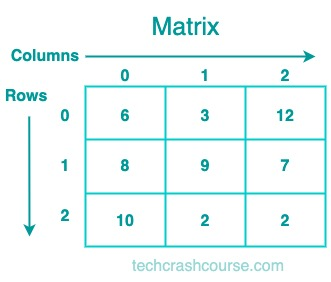# Matrix Data Structure# Matrix Data Structure

A Matrix is a two-dimensional array of elements. It is represented as a collection of rows and columns. A matrix can be used to represent many kinds of data, such as images, graphs, and tables.

## Representation of a Matrix

A matrix can be represented as a two-dimensional array of elements. The number of rows and columns in the matrix is specified when it is created. The elements of the matrix can be of any data type, such as integers, floats, or characters.

Here is an example of a 3x3(3 rows and 3 columns) matrix of integers:## Uses of Matrix Data Structure

Matrices can be used to represent many kinds of data, such as:

• Images: A matrix can be used to represent an image, where each element in the matrix represents a pixel in the image.
• Graphs: A matrix can be used to represent a graph, where each row and column in the matrix represents a vertex in the graph, and the element at position (i,j) represents the weight of the edge between vertex i and vertex j.
• Tables: A matrix can be used to represent a table of data, where each row represents a record and each column represents a field.

• Matrix provide a simple and efficient way to store and manipulate two-dimensional data.

• Matrix can be used to represent many kinds of data.

• A Matrix require a fixed amount of memory, which can be wasteful if the matrix is large and sparse.

• Accessing elements in a matrix can be slow, especially if the matrix is large.

## Matrix Implementation Program

```#include <stdio.h>

#define ROWS 3
#define COLS 3

int main() {
int matrix[ROWS][COLS];
int i, j;

// get input from user
printf("Enter matrix elements:\n");
for (i = 0; i < ROWS; i++) {
for (j = 0; j < COLS; j++) {
scanf("%d", &matrix[i][j]);
}
}

// print the matrix
printf("\nThe matrix is:\n");
for (i = 0; i < ROWS; i++) {
for (j = 0; j < COLS; j++) {
printf("%d ", matrix[i][j]);
}
printf("\n");
}

// calculate the sum of diagonal elements
int sum = 0;
for (i = 0; i < ROWS; i++) {
for (j = 0; j < COLS; j++) {
if (i == j) {
sum += matrix[i][j];
}
}
}

// print the sum of diagonal elements
printf("\nThe sum of diagonal elements is: %d\n", sum);

return 0;
}
```
Output
```Enter matrix elements:
1 2 3
4 5 6
7 8 9

The matrix is:
1 2 3
4 5 6
7 8 9

The sum of diagonal elements is: 15
```

In this program, we first define the number of rows and columns using #define. We then declare a two-dimensional array matrix of size ROWS x COLS. We use nested loops to get input from the user and to print the matrix. We also use another nested loop to calculate the sum of diagonal elements. Finally, we print the sum of diagonal elements.